# Simultaneous Stabilization Using Robust Control

This example uses the Robust Control Toolbox™ commands `ucover` and musyn to design a high-performance controller for a family of unstable plants.

### Plant Uncertainty

The nominal plant model consists of a first-order unstable system.

`Pnom = tf(2,[1 -2]);`

The family of perturbed plants are variations of `Pnom`. All plants have a single unstable pole but the location of this pole varies across the family.

```p1 = Pnom*tf(1,[.06 1]); % extra lag p2 = Pnom*tf([-.02 1],[.02 1]); % time delay p3 = Pnom*tf(50^2,[1 2*.1*50 50^2]); % high frequency resonance p4 = Pnom*tf(70^2,[1 2*.2*70 70^2]); % high frequency resonance p5 = tf(2.4,[1 -2.2]); % pole/gain migration p6 = tf(1.6,[1 -1.8]); % pole/gain migration```

### Covering the Uncertain Model

For feedback design purposes, we need to replace this set of models with a single uncertain plant model whose range of behaviors includes `p1` through `p6`. This is one use of the command `ucover`. This command takes an array of LTI models `Parray` and a nominal model `Pnom` and models the difference `Parray-Pnom` as multiplicative uncertainty in the system dynamics.

Because `ucover` expects an array of models, use the `stack` command to gather the plant models `p1` through `p6` into one array.

`Parray = stack(1,p1,p2,p3,p4,p5,p6);`

Next, use `ucover` to "cover" the range of behaviors `Parray` with an uncertain model of the form

```P = Pnom * (1 + Wt * Delta) ```

where all uncertainty is concentrated in the "unmodeled dynamics" `Delta` (a `ultidyn` object). Because the gain of `Delta` is uniformly bounded by 1 at all frequencies, a "shaping" filter `Wt` is used to capture how the relative amount of uncertainty varies with frequency. This filter is also referred to as the uncertainty weighting function. Try a 4th-order filter `Wt` for this example:

```orderWt = 4; Parrayg = frd(Parray,logspace(-1,3,60)); [P,Info] = ucover(Parrayg,Pnom,orderWt,'InputMult');```

The resulting model `P` is a single-input, single-output uncertain state-space (USS) object with nominal value `Pnom`.

`P`
```P = Uncertain continuous-time state-space model with 1 outputs, 1 inputs, 5 states. The model uncertainty consists of the following blocks: Parrayg_InputMultDelta: Uncertain 1x1 LTI, peak gain = 1, 1 occurrences Type "P.NominalValue" to see the nominal value, "get(P)" to see all properties, and "P.Uncertainty" to interact with the uncertain elements. ```
`tf(P.NominalValue)`
```ans = 2 ----- s - 2 Continuous-time transfer function. ```

A Bode magnitude plot confirms that the shaping filter `Wt` "covers" the relative variation in plant behavior. As a function of frequency, the uncertainty level is 30% at 5 rad/sec (-10dB = 0.3) , 50% at 10 rad/sec, and 100% beyond 29 rad/sec.

```Wt = Info.W1; bodemag((Pnom-Parray)/Pnom,'b--',Wt,'r'); grid title('Relative Gaps vs. Magnitude of Wt')```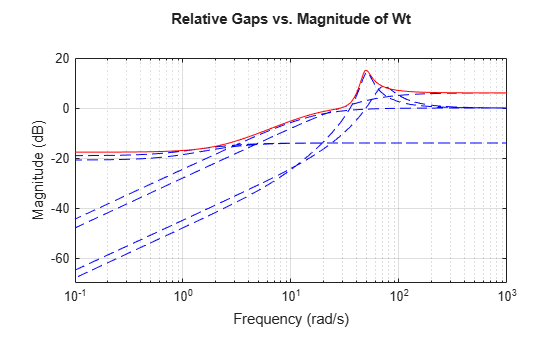### Creating the Open-loop Design Model

To design a robust controller for the uncertain plant model `P`, we choose a desired closed-loop bandwidth and minimize the sensitivity to disturbances at the plant output. The control structure is shown below. The signals `d` and `n` are the load disturbance and measurement noise. The controller uses a noisy measurement of the plant output `y` to generate the control signal `u`.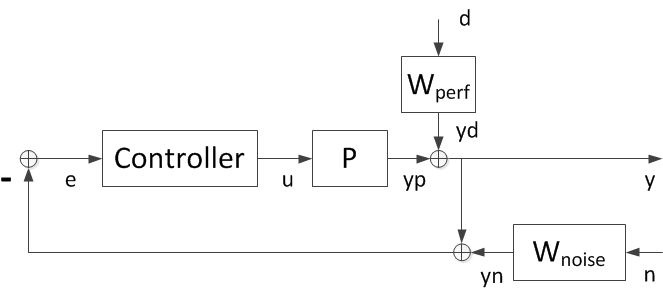Figure 1: Control Structure.

The filters `Wperf` and `Wnoise` are selected to enforce the desired bandwidth and some adequate roll-off. The closed-loop transfer function from `[d;n]` to `y` is

``` y = [Wperf * S , Wnoise * T] [d;n] ```

where `S=1/(1+PC)` and `T=PC/(1+PC)` are the sensitivity and complementary sensitivity functions. If we design a controller that keeps the closed-loop gain from `[d;n]` to `y` below 1, then

``` |S| < 1/|Wperf| , |T| < 1/|Wnoise| ```

By choosing appropriate magnitude profiles for `Wperf` and `Wnoise`, we can enforce small sensitivity (`S`) inside the bandwidth and adequate roll-off (`T`) outside the bandwidth.

For example, choose `Wperf` as a first-order low-pass filter with a DC gain of 500 and a gain crossover at the desired bandwidth `desBW`:

```desBW = 4.5; Wperf = makeweight(500,desBW,0.33); tf(Wperf)```
```ans = 0.33 s + 4.248 -------------- s + 0.008496 Continuous-time transfer function. ```

Similarly, pick `Wnoise` as a second-order high-pass filter with a magnitude of 1 at `10*desBW`. This will force the open-loop gain `PC` to roll-off with a slope of -2 for frequencies beyond `10*desBW`.

```NF = (10*desBW)/20; % numerator corner frequency DF = (10*desBW)*50; % denominator corner frequency Wnoise = tf([1/NF^2 2*0.707/NF 1],[1/DF^2 2*0.707/DF 1]); Wnoise = Wnoise/abs(freqresp(Wnoise,10*desBW))```
```Wnoise = 0.1975 s^2 + 0.6284 s + 1 ------------------------------ 7.901e-05 s^2 + 0.2514 s + 400 Continuous-time transfer function. ```

Verify that the bounds `1/Wperf` and `1/Wnoise` on `S` and `T` do enforce the desired bandwidth and roll-off.

```bodemag(1/Wperf,'b',1/Wnoise,'r',{1e-2,1e3}), grid title('Performance and roll-off specifications') legend('Bound on |S|','Bound on |T|','Location','NorthEast')```Next use `connect` to build the open-loop interconnection (block diagram in Figure 1 without the controller block). Specify each block appearing in Figure 1, name the signals coming in and out of each block, and let `connect` do the wiring:

```P.u = 'u'; P.y = 'yp'; Wperf.u = 'd'; Wperf.y = 'Wperf'; Wnoise.u = 'n'; Wnoise.y = 'Wnoise'; S1 = sumblk('e = -ym'); S2 = sumblk('y = yp + Wperf'); S3 = sumblk('ym = y + Wnoise'); G = connect(P,Wperf,Wnoise,S1,S2,S3,{'d','n','u'},{'y','e'});```

`G` is a 3-input, 2-output uncertain system suitable for robust controller synthesis with musyn.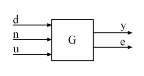### Robust Controller Synthesis

The design is carried out with the automated robust design command musyn. The target bandwidth is 4.5 rad/s.

```ny = 1; nu = 1; [C,muPerf] = musyn(G,ny,nu);```
```D-K ITERATION SUMMARY: ----------------------------------------------------------------- Robust performance Fit order ----------------------------------------------------------------- Iter K Step Peak MU D Fit D 1 353.6 249.5 251.9 0 2 70.74 9.964 10.05 4 3 1.98 1.604 1.621 8 4 1.164 1.164 1.188 10 5 1.089 1.089 1.098 10 6 1.05 1.05 1.055 10 7 1.027 1.027 1.032 8 8 1.016 1.016 1.023 8 9 1.013 1.013 1.02 8 10 1.011 1.011 1.013 8 Best achieved robust performance: 1.01 ```

When the robust performance indicator `muPerf` is near 1, the controller achieves the target closed-loop bandwidth and roll-off. As a rule of thumb, if `muPerf` is less than 0.85, then the performance can be improved upon, and if `muPerf` is greater than 1.2, then the desired closed-loop bandwidth is not achievable for the specified plant uncertainty.

Here `muPerf` is approximately 1 so the objectives are met. The resulting controller `C` has 18 states:

`size(C)`
```State-space model with 1 outputs, 1 inputs, and 16 states. ```

You can use the `reduce` and musyn`perf` commands to simplify this controller. Compute approximations of orders 1 through 17.

```NxC = order(C); Cappx = reduce(C,1:NxC);```

For each reduced-order controller, use musyn`perf` to compute the robust performance indicator and compare it with `muPerf`. Keep the lowest-order controller with performance no worse than 1.05 * `muPerf`, a performance degradation of 5% or less.

```for k=1:NxC Cr = Cappx(:,:,k); % controller of order k bnd = musynperf(lft(G,Cr)); if bnd.UpperBound < 1.05 * muPerf break % abort with the first controller meeting the performance goal end end order(Cr)```
```ans = 6 ```

This yields a 6th-order controller `Cr` with comparable performance. Compare `Cr` with the full-order controller `C`.

```opt = bodeoptions; opt.Grid = 'on'; opt.PhaseMatching = 'on'; bodeplot(C,'b',Cr,'r--',opt) legend('Full-order C','Reduced-order Cr','Location','NorthEast')```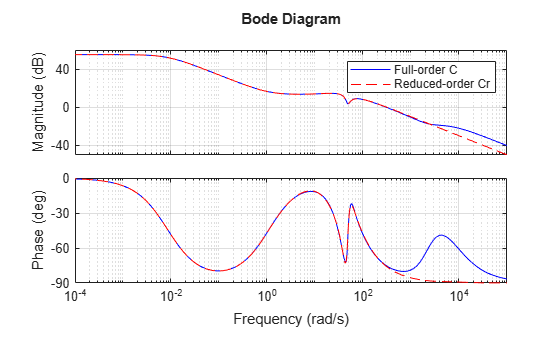### Robust Controller Validation

Plot the open-loop responses of the plant models `p1` through `p6` with the simplified controller `Cr`.

`bodeplot(Parray*Cr,'g',{1e-2,1e3},opt);`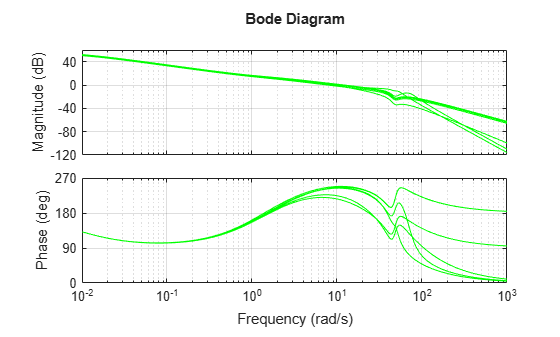Plot the responses to a step disturbance at the plant output. These are consistent with the desired closed-loop bandwidth and robust to the plant variations, as expected from a Robust Performance mu-value of approximately 1.

`step(feedback(1,Parray*Cr),'g',10/desBW);`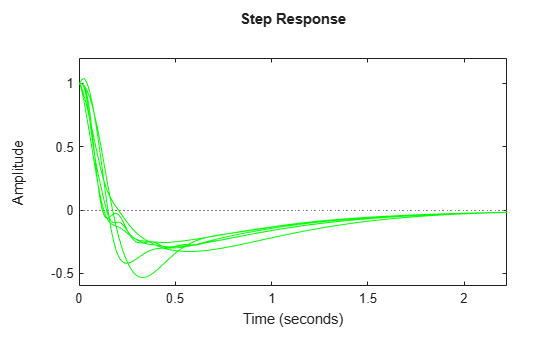### Varying the Target Closed-Loop Bandwidth

The same design process can be repeated for different closed-loop bandwidth values `desBW`. Doing so yields the following results:

• Using `desBW` = 8 yields a good design with robust performance `muPerf` of 1.09. The step responses across the `Parray` family are consistent with a closed-loop bandwidth of 8 rad/s.

• Using `desBW` = 20 yields a poor design with robust performance `muPerf` of 1.35. This is expected because this target bandwidth is in the vicinity of very large plant uncertainty. Some of the step responses for the plants `p1,...,p6` are actually unstable.

• Using `desBW` = 0.3 yields a poor design with robust performance `muPerf` of 2.2. This is expected because `Wnoise` imposes roll-off past 3 rad/s, which is too close to the natural frequency of the unstable pole (2 rad/s). In other words, proper control of the unstable dynamics requires a higher bandwidth than specified.